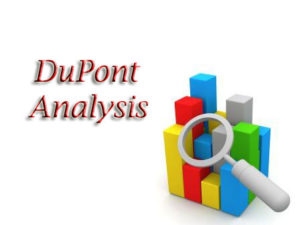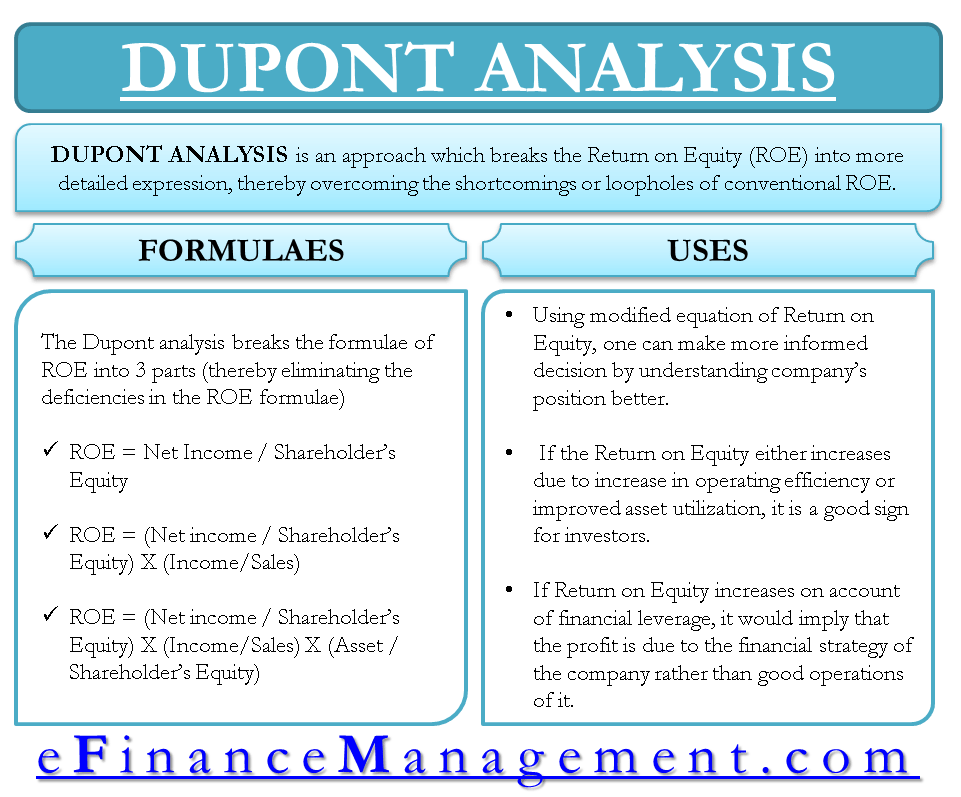# DuPont Analysis

DuPont Analysis is an approach which breaks the Return on Equity (ROE) into more detailed expression, thereby overcoming the shortcomings or loopholes of conventional ROE. It was developed by DuPont Corporation in the 1920s. DuPont analysis interprets the basic ROE ratio in a manner so that it provides a great insight into the performance of the company.

## How to Calculate ROE?

It is the profit that a company derives for every dollar invested by the shareholders in the company. ROE or Return on Equity is given by:

 Net Income Return on Equity (ROE) = —————— Shareholder’s Equity

Net Income = Net profit derived from the business

Shareholders’ Equity = Capital invested by shareholders of the company

ROE is a simple ratio and requires only 2 figures to calculate viz. Net Income and Shareholders Equity. Higher this ratio, better are the returns that shareholders get for every dollar invested. So, ideally from an investor’s point of view, Return on Equity should be as high as possible.ROE focuses only on the returns of the equity shareholders. It only measures a percentage rate of return earned by the investors from the underlying company. Therefore, the scope of this ratio is quite narrow. On the contrary, the ratio is converted into an expression by DuPont which not only presents the returns earned by the investors but also talks about three important parameters about a company’s performance.  These three parameters are Profitability, Operating Efficiency / Asset Utilization, and Financial Leverage.## Formula of DuPont Analysis

DuPont Analysis overcomes this drawback of ROE. It breaks ROE into 3 parts; thereby one can analyze the reason for increase/decrease in ROE.

 Net Income ROE = —————— Shareholder’s Equity

Multiplying equation by sales/sales

 Net Sales Income ROE = ————- * ——- Shareholder’s Equity Sales

Again multiplying the above equation by assets/assets

 Net Income Sales Assets ROE = ————- * ——- * ————- Sales Assets Shareholder’s Equity

The above equation for ROE is broken down into 3 components:

1. Net Income / Sales = Operating Efficiency (as measured by profit margin).
2. Sales / Assets = Asset Utilization (as measured by total asset turnover).
3. Assets / Shareholders’ Equity = Financial leverage (as measured by the equity multiplier).

## Use of DuPont Analysis

Using the modified equation of Return on Equity, one can make a more informed decision and understand the company position better. If the Return on Equity either increases due to increase in operating efficiency or improved asset utilization, it is a good sign for investors. It would imply that either company is making higher margins on its sales or the company is making better use of its assets or both.

On the other hand, if the Return on Equity increases on account of financial leverage, it would imply that the profit is due to the financial strategy of the company rather than good operations of it. Too higher a debt ratio also makes a company riskier proposal for investment.

In essence, a manager should try increasing the ROE by way of either higher operating efficiency or better asset utilization. There is no reservation for using the third parameter also i.e. financial leverage, but it will increase the risk of bankruptcy in case the lenders ask for the large sum of money.

## Example Explaining DuPont Analysis

Consider a hypothetical scenario of companies X and Y with below numbers.

 Particulars X Y 1. Operating Profit Margin Ratio 0.20 0.12 2. Asset Turnover Ratio 0.30 0.30 3. Financial Leverage or Equity Multiplier 2.00 3.33 ROE (1*2*3) 0.12 0.12

ROE for both the companies is same viz. 12%. But company X has better Operating margin as compared to company Y. Hence, X can be considered as a safer option to invest in as compared to Y.

Last updated on : April 30th, 2019
What’s your view on this? Share it in comments below.